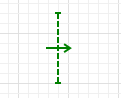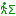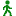AnyLogic

# Ped Flow StatisticsPed Flow Statistics is a graphical markup element that collects statistics on pedestrians crossing the drawn cross-section.

You draw its line on animation, and it will collect statistics on the number of pedestrians crossing this particular line. For example, you can draw the element’s line across a corridor, and it will count statistics on pedestrians passing through this corridor.

You can count pedestrians crossing the line either in one particular direction or in both directions (this is configured by the element parameters).

Using the element’s traffic() and intensity() functions, you can know the pedestrian traffic and intensity value correspondingly.

Traffic is calculated as the number of pedestrians that crossed the line in the specified direction recently, see the Functions section for details.

Intensity is calculated as the traffic value divided by the line length (in meters) and is measured in pedestrians/(hour*meter).

Demo model: Pedestrian Flow Statistics

To add Ped Flow Statistics line on the graphical diagram

1. Drag thePed Flow Statistics element from the Space Markup section of thePedestrian Library palette to the graphical diagram.
2. Place the line as you need by moving its end point with the mouse.

## Properties

General

Name — The name of the shape. The name is used to identify and access the shape from code.

Ignore — If selected, the shape is excluded from the model.

Visible on upper agent — If selected, the shape is also visible on the upper agent where this agent lives.

Visible — Here you specify whether the shape is visible on animation at model runtime, or not. Using the control, choose yes or no.

Direction — Here you can choose the pedestrian movement direction that will be considered while collecting the statistics. You may collect statistics for pedestrians crossing the element’s line only to the Left, only to the Right, or crossing it in Both directions. The arrow indicating the chosen direction is drawn in graphical editor to simplify the things for the modeler.

Line color — The line color.

Position and size

Level — The level this line belongs to. Statistics will be collected on all pedestrians crossing the element’s line in the specified Direction on this level.

X — X-coordinate of the line (its start point).

Y — Y-coordinate of the line (its start point).

dX — X-offset of the end point regarding the start point of the line.

dY — Y-offset of the end point regarding the start point of the line.

Show in — Here you can choose whether you want the shape to be shown both In 2D and 3D animation, or in 2D only, or in 3D only.

Show name — If selected, the shape’s name is displayed on the graphical diagram.

## Functions

Flow statistics
Function Description
long countPeds() Returns the total number of pedestrians passed through the line.
double traffic() Returns the pedestrian flow traffic value, in pedestrians/hour. The traffic value is calculated as the number of pedestrians that crossed the cross section line in the specified direction (set by the element’s parameters) during the last five minutes (in terms of model time). To get the value in pedestrians/hour, the observed number of pedestrians is multiplied by 12.
During the warm-up period (the first five minutes of the simulation and first five minutes after the statistics reset), traffic statistics is collected as well. However, the same formula (observed number of pedestrians multiplied by 12) is used regardless the actually shorter statistics collection period.
double intensity() Returns the pedestrian flow intensity, in pedestrians/(hour*meter). The intensity is calculated as the traffic value divided by the line length (in meters).
void reset() Resets the traffic and intensity values collected by this element.
Flow direction
Function Description
FlowStatisticsDirection getDirection() Returns the current flow direction, for which this element collects statistics.

Valid values:
FLOW_STATISTICS_LEFT
FLOW_STATISTICS_RIGHT
FLOW_STATISTICS_BOTH
void setDirection(FlowStatisticsDirection direction) Sets the flow direction, for which this element will collect statistics.

direction — the new direction.

Valid values:
FLOW_STATISTICS_LEFT
FLOW_STATISTICS_RIGHT
FLOW_STATISTICS_BOTH
Color
Function Description
Color getColor() Returns the color of the shape.
void setColor(Color color) Sets the color of the shape.

color — the new color
Visibility
Function Description
boolean isVisible() Returns true if the shape is visible; returns false otherwise.
void setVisible(boolean v) Sets the visibility of the shape.

v — visibility. If v is true — the shape is set to be visible, if it is false — not visible.
Level
Function Description
Level getLevel() Returns the level this space markup shape belongs to.